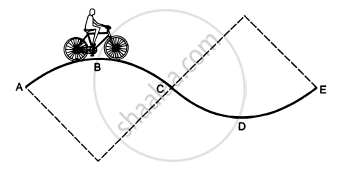Share

# A Track Consists of Two Circular Parts Abc and Cde of Equal Radius 100 M and Joined Smoothly as Shown in Figure. Each Part Subtends a Right Angle at Its Centre. - Physics

ConceptCircular Motion

#### Question

A track consists of two circular parts ABC and CDE of equal radius 100 m and  joined smoothly as shown in figure. Each part subtends a right angle at its centre. A cycle weighing 100 kg together with the rider travels at a constant speed of 18 km/h on the track. (a) Find the normal contact force by the road on the cycle when it is at B and at D. (b) Find the force of friction exerted by the track on the tyres when the cycle is at B, C and. (c) Find the normal force between the road and the cycle just before and just after the cycle crosses C. (d) What should be the minimum friction coefficient between the road and the tyre, which will ensure that the cyclist can move with constant speed? Take g = 10 m/s2.#### Solution

Given:
Radius of the curves = r = 100 m
Mass of the cycle = m = 100 kg
Velocity = v = 18 km/hr = 5 m/s

$(a) \text {At B}, \text {we have :}$

$\text{mg }- \frac{m v^2}{r} = N$

$\Rightarrow N = (100 \times 10) - \left( 100 \times \frac{25}{100} \right)$

$= 1000 - 25 = 975 N$

$\text {At D}, \text {we have : }$

(b) At B and D, we have:
Tendency of the cycle to slide is zero.
So, at B and D, frictional force is zero.
At C, we have :
mgsinθ = f

$\Rightarrow 1000 \times \left( \frac{1}{\sqrt{2}} \right) = 707 N$

$(c) \text { i Before }C,$

$\text{mg}\cos\theta - N = \frac{m v^2}{r}$

$\Rightarrow N = \text{mg} \cos\theta - \frac{m v^2}{r}$

$= 707 - 25 = 682 N$

ii$\ \text{N - mg}\cos\theta = \frac{m v^2}{r}$

$\Rightarrow N = \frac{m v^2}{r} + \text{mg}\cos\theta$

$= 25 + 707 = 732 N$

(d) To find the minimum coefficient of friction, we have to consider a point where N is minimum or a point just before c .

$\text { Therefore, we have : }$

$\mu N = mg\sin\theta$

$\Rightarrow \mu \times 682 = 707$

$\Rightarrow \mu = 1 . 037$

Is there an error in this question or solution?

#### APPEARS IN

Solution A Track Consists of Two Circular Parts Abc and Cde of Equal Radius 100 M and Joined Smoothly as Shown in Figure. Each Part Subtends a Right Angle at Its Centre. Concept: Circular Motion.
S